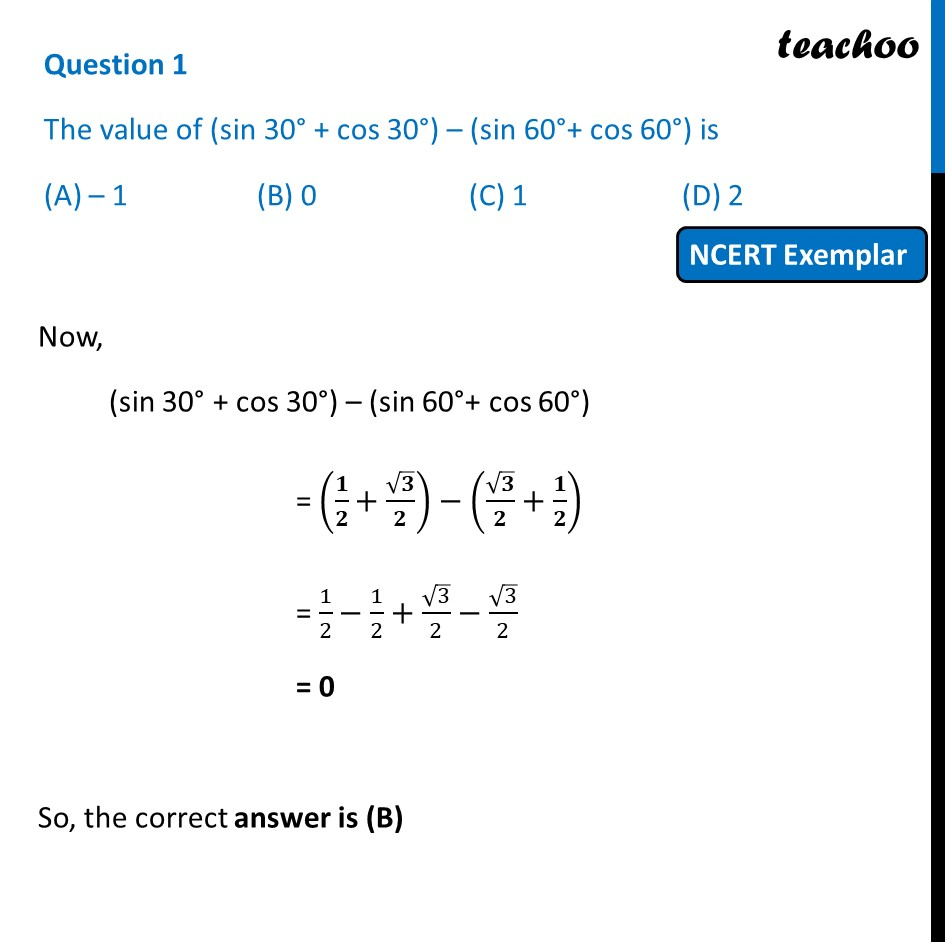## (D) 21. Chapter 8 Class 10 Introduction to Trignometry (Term 1)
2. Serial order wise
3. NCERT Exemplar - MCQ

Transcript

Question 1 The value of (sin 30° + cos 30°) – (sin 60°+ cos 60°) is (A) – 1 (B) 0 (C) 1 (D) 2 Now, (sin 30° + cos 30°) – (sin 60°+ cos 60°) = (𝟏/𝟐+√𝟑/𝟐)−(√𝟑/𝟐+𝟏/𝟐) = 1/2−1/2+√3/2−√3/2 = 0 So, the correct answer is (B)